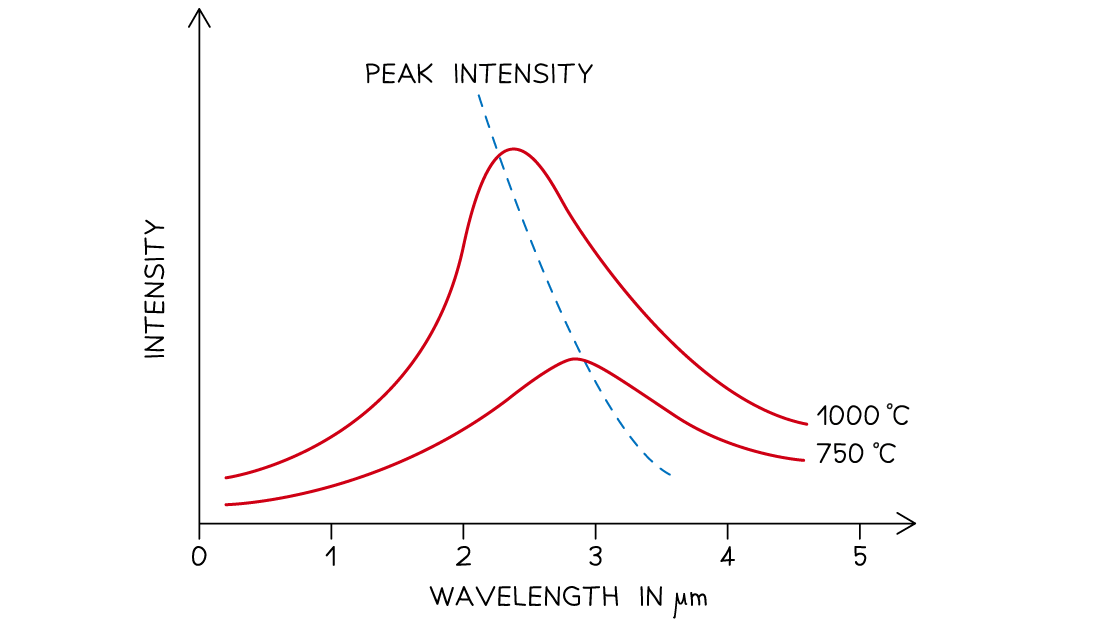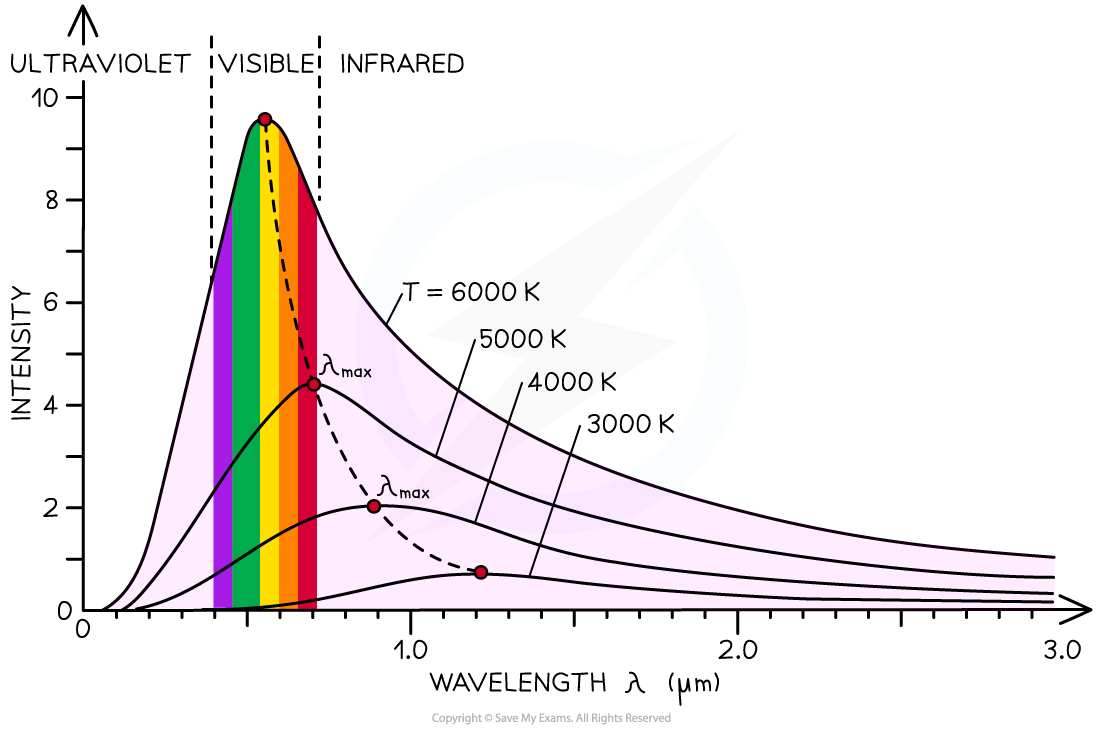# IB DP Physics: SL复习笔记8.2.2 Black-Body Radiation

• Black body radiation is the name given to the thermal radiation emitted by all bodies (objects)
• All objects, no matter what temperature, emit black body radiation in the form of electromagnetic waves
• These electromagnetic waves usually lie in the infrared region of the spectrum
• Black-body radiation can also be emitted in the form of visible light or other wavelengths, depending on the temperature
• The hotter object, the more infrared radiation it radiates in a given time
• A perfect black body is defined as:

An object that absorbs all of the radiation incident on it and does not reflect or transmit any radiation

• Since a good absorber is also a good emitter, a perfect black body would be the best possible emitter too
• As a result, an object which perfectly absorbs all radiation will be black
• This is because the colour black is what is seen when all colours from the visible light spectrum are absorbed
• The intensity and wavelength distribution of any emitted waves depends on the temperature of the body
• This is represented on a black body radiation curve
• As the temperature increases, the peak of the curve moves
• This moves to a lower wavelength and a higher intensityBlack body spectrum for objects of different temperatures

• From the electromagnetic spectrum, waves with a smaller wavelength have higher energy (e.g. UV rays, X-rays)
• When an object gets hotter, the amount of thermal radiation it emits increases
• This increases the thermal energy emitted and therefore the wavelength of the emitted radiation decreases
• At room temperature objects emit thermal radiation in the infrared region of the spectrum
• At around 1000 °C an object will emit a significant amount of red light
• At 6000 °C an object will mainly emit white or blue light (and some ultraviolet)
• At even higher temperatures objects will emit ultraviolet or even X-rays
• A black body is an object which:
• Absorbs all the radiation that falls on it, and is also a good emitter
• Does not reflect or transmit any radiation
• A black body is a theoretical object, however, stars are the best approximation there is
• The radiation emitted from a black body has a characteristic spectrum that is determined by the temperature aloneThe intensity-wavelength graph shows how thermodynamic temperature links to the peak wavelength for four different bodies

### Stefan–Boltzmann Law & Wien’s Displacement Law

#### Wien’s Displacement Law

• Wien’s displacement law relates the wavelength emitted by a body to its surface temperature, it states:

The black body radiation curve for different temperatures peaks at a wavelength which is inversely proportional to the temperature

• This relation can be written as:• The full equation for Wien's Law is given by

λmaxT = 2.9 × 10−3 m K

• Where:
• λmax = peak wavelength emitted by the body (m)
• T = temperature of the body (K)
• This equation shows:
• The higher the temperature of a body, the shorter the wavelength it emits at the peak intensity
• The higher the temperature of a body, the greater the intensity of the radiation at each wavelength

#### Stefan–Boltzmann Law

• The power output of a black body depends on two factors:
• Its surface temperature
• The relationship between these is known as the Stefan-Boltzmann Law, which states:

The total energy emitted by a black body per unit area per second is proportional to the fourth power of the absolute temperature of the body

• It is equal to:

P = σAT4

• Where:
• P = total power emitted by the black body (W)
• σ = the Stefan-Boltzmann constant
• A = total surface area of the black body (m2)
• T = absolute temperature of the body (K)
• When considering a sphere (such as a star) the surface area is equal to 4πr2
• In this case, the Stefan-Boltzmann law can be written as:

P = 4πr2σT4

#### Worked Example

Betelguese is our nearest red giant star. It has a power output of 4.49 × 1031 W and emits radiation with a peak wavelength of 850 nm.
Calculate the ratio of the radius of Betelgeuse rB to the radius of the Sun rs.
Radius of the sun rs = 6.95 × 108 m.

Step 1: Write down Wien’s displacement law

λmaxT = 2.9 × 10−3 m K

Step 2: Rearrange Wien’s displacement law to find the surface temperature of BetelgueseStep 3: Write down the Stefan-Boltzmann law

P = σAT4

P = 4πr2σT4

Step 4: Rearrange for r and calculate the stellar radius of BetelgueseStep 5: Calculate the ratio rB / rs• Therefore, the radius of Betelguese is about 1000 times larger than the Sun’s radius